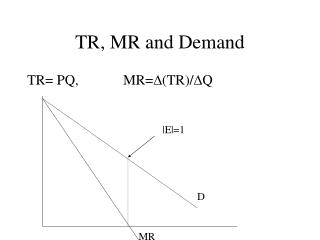Download PresentationTR, MR and Demand# TR, MR and Demand - PowerPoint PPT Presentation

Download Presentation##### TR, MR and Demand

Download Policy: Content on the Website is provided to you AS IS for your information and personal use and may not be sold / licensed / shared on other websites without getting consent from its author. While downloading, if for some reason you are not able to download a presentation, the publisher may have deleted the file from their server.

- - - - - - - - - - - - - - - - - - - - - - - - - - - E N D - - - - - - - - - - - - - - - - - - - - - - - - - - -
##### Presentation Transcript

1. TR, MR and Demand TR= PQ, MR=D(TR)/DQ |E|=1 D MR

2. Production and Costsan Economist’s view Q = f ( L, K,….) Short-Run (production): K – fixed Long-Run (planning): everything is flexible

3. Measures of productivity • Total product (TP) TP = Q (L, K) = L1/2 K1/2 Fixed vs variable proportion functions • Average Product (AP) APL = Q/L APK = Q/K • Marginal Product (MP) MPL = DQ/DL

4. Law of Diminishing Marginal Product • Seen in the slope of the MP curve • More intense usage of fixed input by the variable inputs may initially increase Q;however, after a certain point inputs are lessproductive and produce less output for each additional input added • Can employ additional inputs when MP is decreasing. Do not employ additional inputs when MP is negative

5. Relationships between MP, AP, and TP If MP>0 then TP is rising If MP<0 then TP is falling If MP is rising then the output function is convex If MP is falling then the output function is concave MP as the slope of the production function If MP>AP then AP is rising If MP<AP then AP is falling

6. Costs of Production fixed vs. variable vs. sunk Opportunity cost (explicit + implicit) Cost of Labor: wage bill User Cost of Capital: Economic Depreciation + Interest Rate * Value of Capital

7. costs TC = w L + r K Variable (w L), fixed (r K) Averages: ATC = TC/Q AVC = TVC/Q AFC = TFC/Q Marginal: MC = DTC/DQ = DTVC/DQ

8. Some cost identities and profit maximization in the short-run • MC=MR • MC = w / MPL • W = VMPL=MR*MPL • AVC = w / APL

9. Long-run costs Everything is variable Isoquant and Isocost analysis & Input substitution

10. Isocostw L + r K = C K C/r -(slope) = (C/r)/(C/w) = w/r C/w L

11. IsoquantQ = f ( L, K ) = constant K -(slope) = (dK/dL) dQ = MPL dL + MPK dK dK/dL = MPL/MPK dK/dL – MRTS of labor for capital Set dL =1 L

12. Equilibriumcost minimization K MPL/MPK = w/r w/MPL = r/MPK the last \$ spent on capital brings the same change in output as the last \$ spent on labor L

13. returns to scale % change in inputs => % change in output (%D output) > (%D inputs) increasing returns Q = KaLb if a + b > 1 then we have increasing returns to scale. economies of scale and the LRAC specialization and technology economies of scope sharing of inputs

14. Cost minimization MPL/MPK = w/r w/MPL = r/MPK the last \$ spent on capital brings the same change in output as the last \$ spent on labor

15. Profit maximization Profit = total revenues – total costs Profits are maximized when MC = MR MC = W/MPL => W = MR * MPL

16. market structure oligopoly mc monopoly Perfect competition

17. Perfect competition and the internet Assumptions: • number of firms • Ease of entry and exit • Perfect information • Identical transaction costs • Homogeneous good Horizontal demand and MR. Shut down and break even price levels Long-run and cost structure of the industry

18. monopoly • Market power & MR • What is Monopoly and why do they exist? natural monopoly barriers to entry (legal, brand loyalty….) is Microsoft a monopoly? Measures of monopoly power • elasticity approach • Learner index (P-MC)/P

19. Monopolistic competition Large number of firms and heterogeneous goods

20. oligopoly Few players and strategic behavior Oligopolies arise because of the same reasons as monopolies. Models for studying Oligopoly Kinked Demand Model (discontinuous MR) Cournot Duopoly Model Game Theory Bertrand and Stackelberg Models

21. Game theory Cooperative vs non-cooperative games Basic 2X2 game framework analysis Price leadership models, airlines Tacit collusion (explicit) Implicit collusions and the MIT case Tree form games and entry deterrence

22. Multi-plant production obtaining total MC Multi-market marketing price discrimination vs price differentiation obtaining total marginal cost curve